# RD Sharma Solutions Class 12 Linear Programming Exercise 30.4

RD Sharma Solutions for Class 12 Maths Chapter 30 Linear Programming Exercise 30.4, is given here to help students excel in their board exams. The solutions are designed by our subject experts, to help understand the concepts and methods to solve problems in a shorter period and also to boost the confidence levels among students. Download the RD Sharma Class 12 Solutions pdf for free from the links given below.

## Download PDF of RD Sharma Solutions for Class 12 Maths Chapter 30 Exercise 4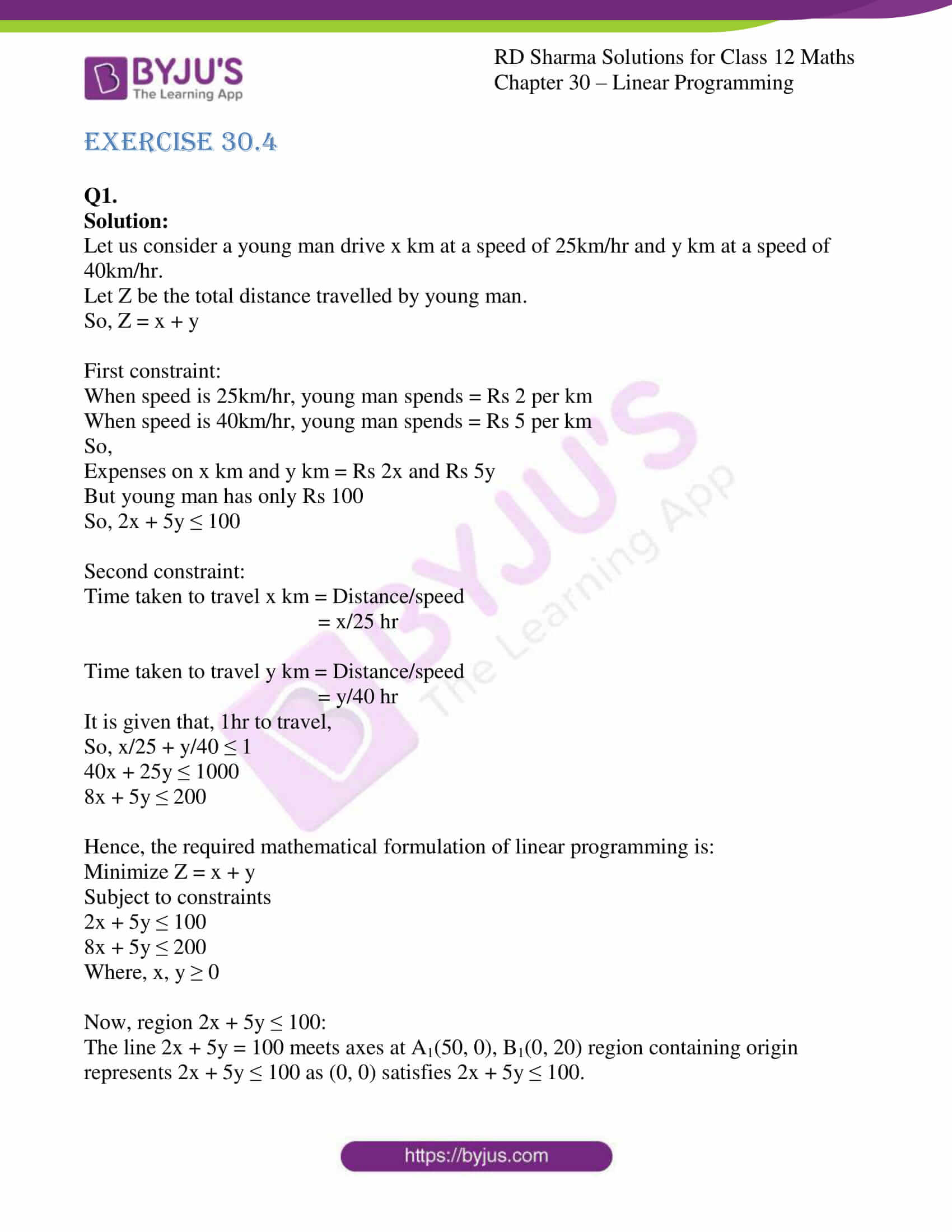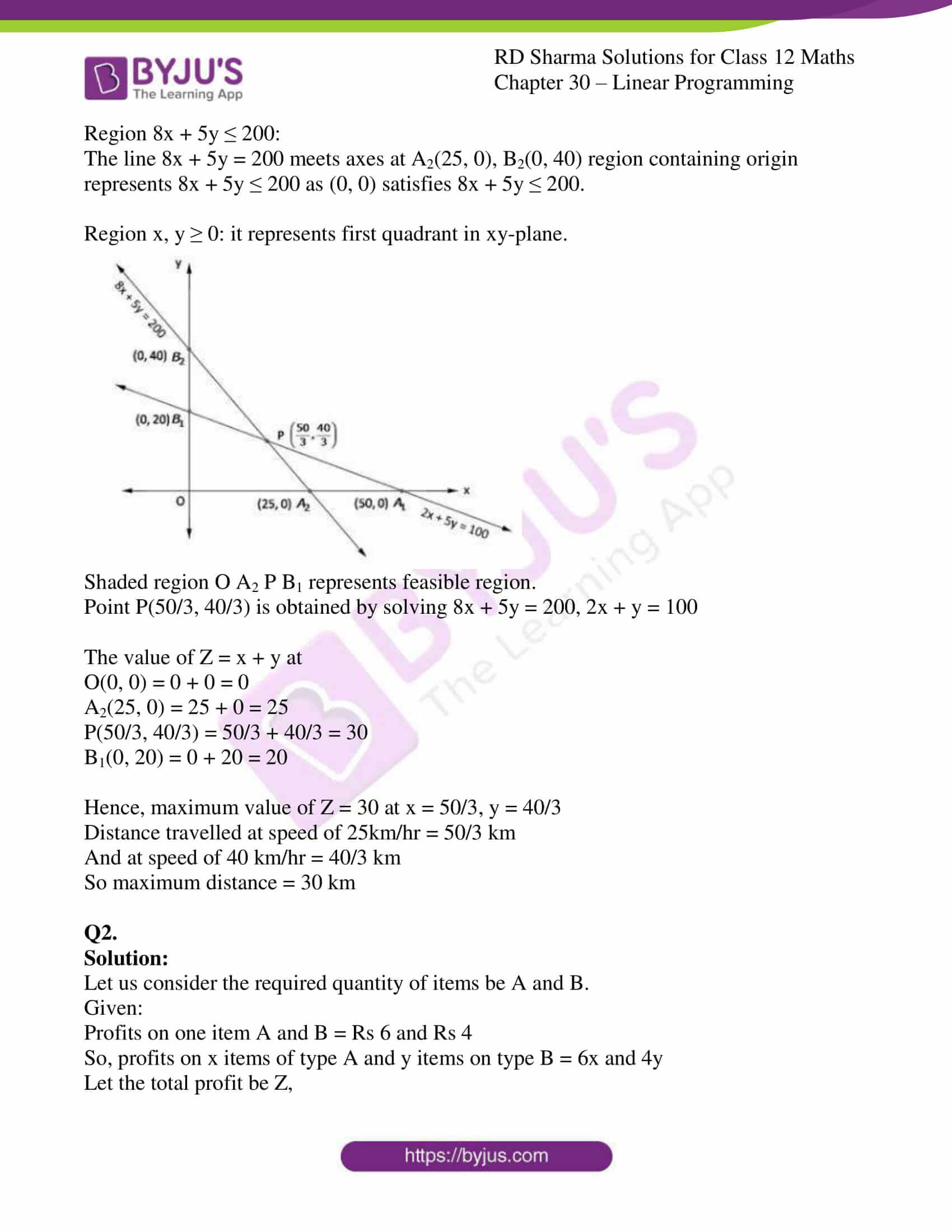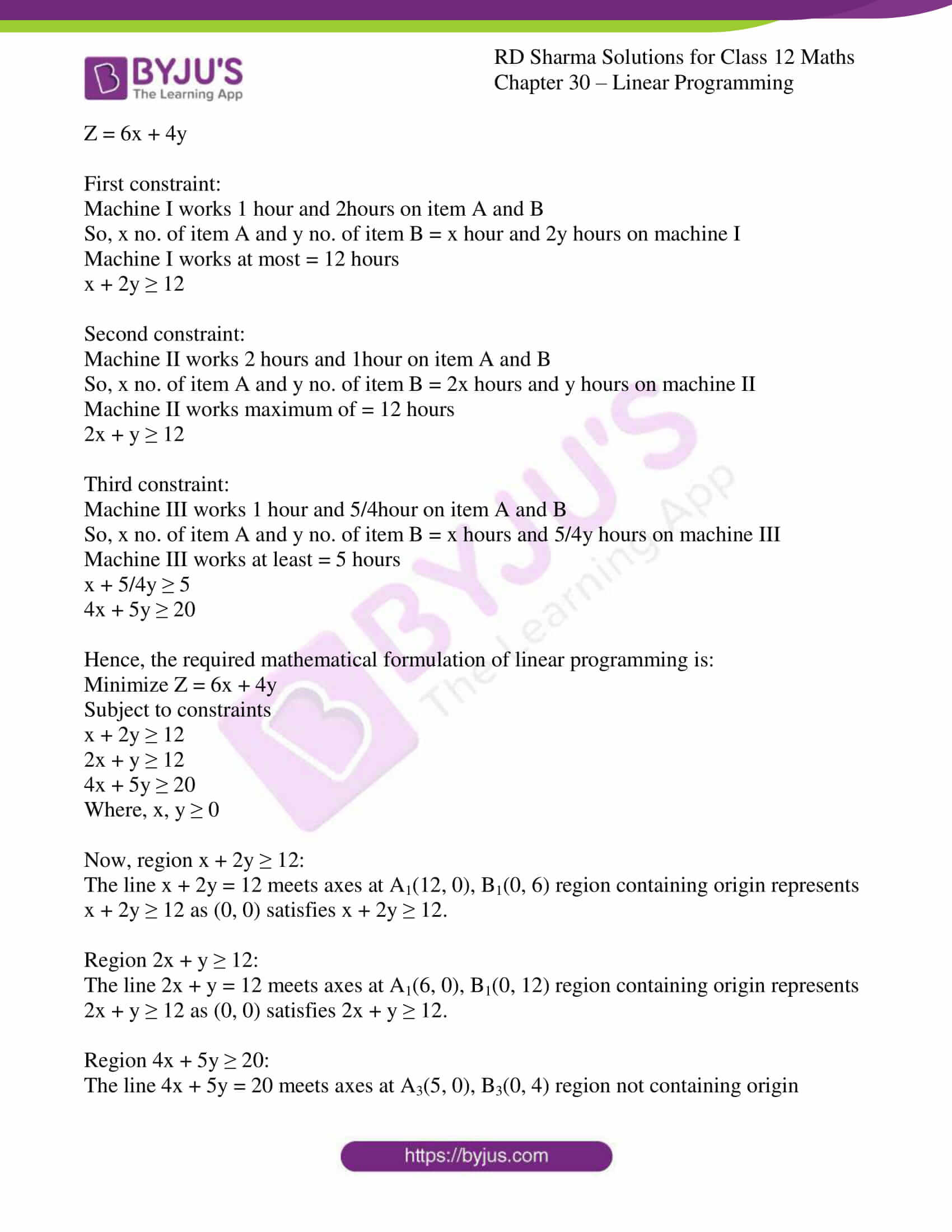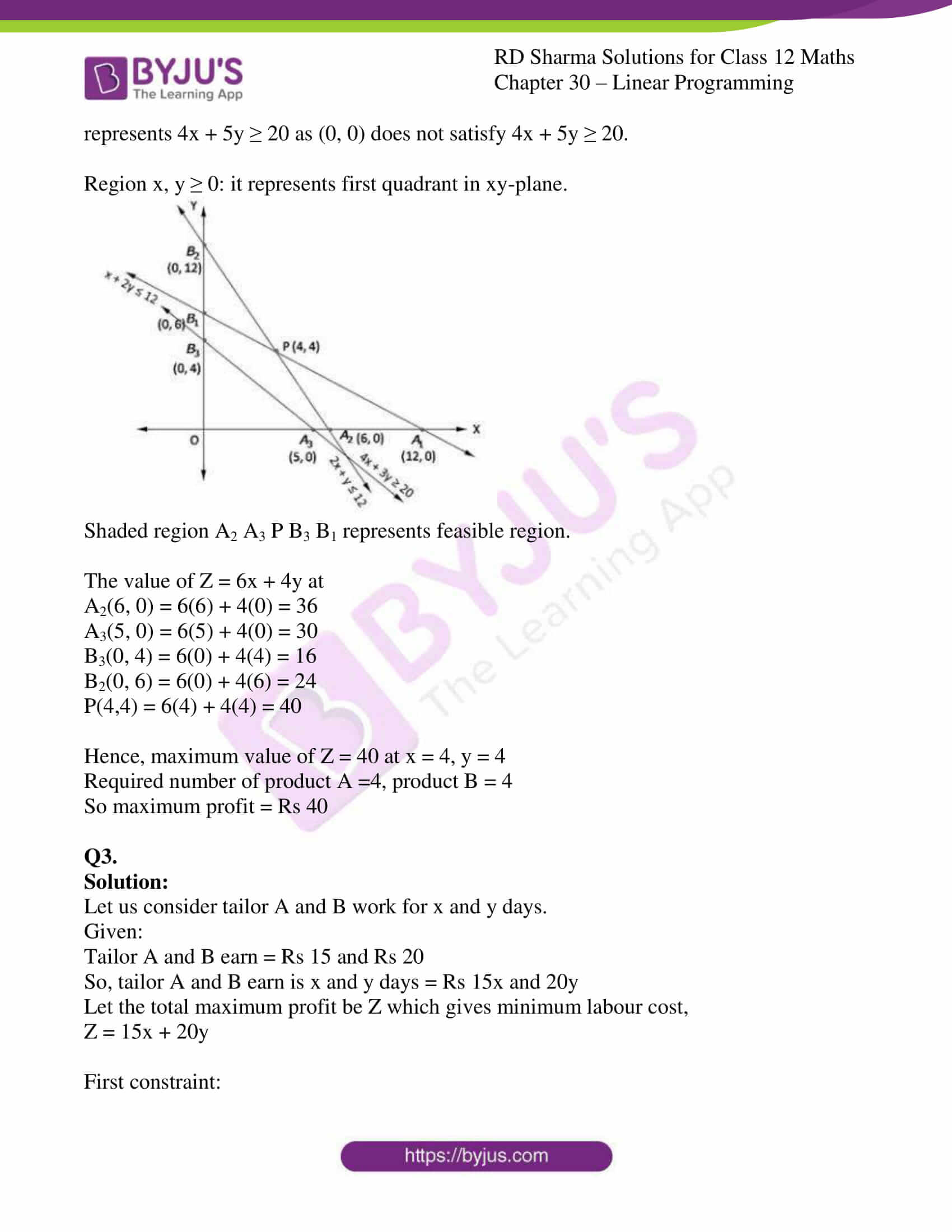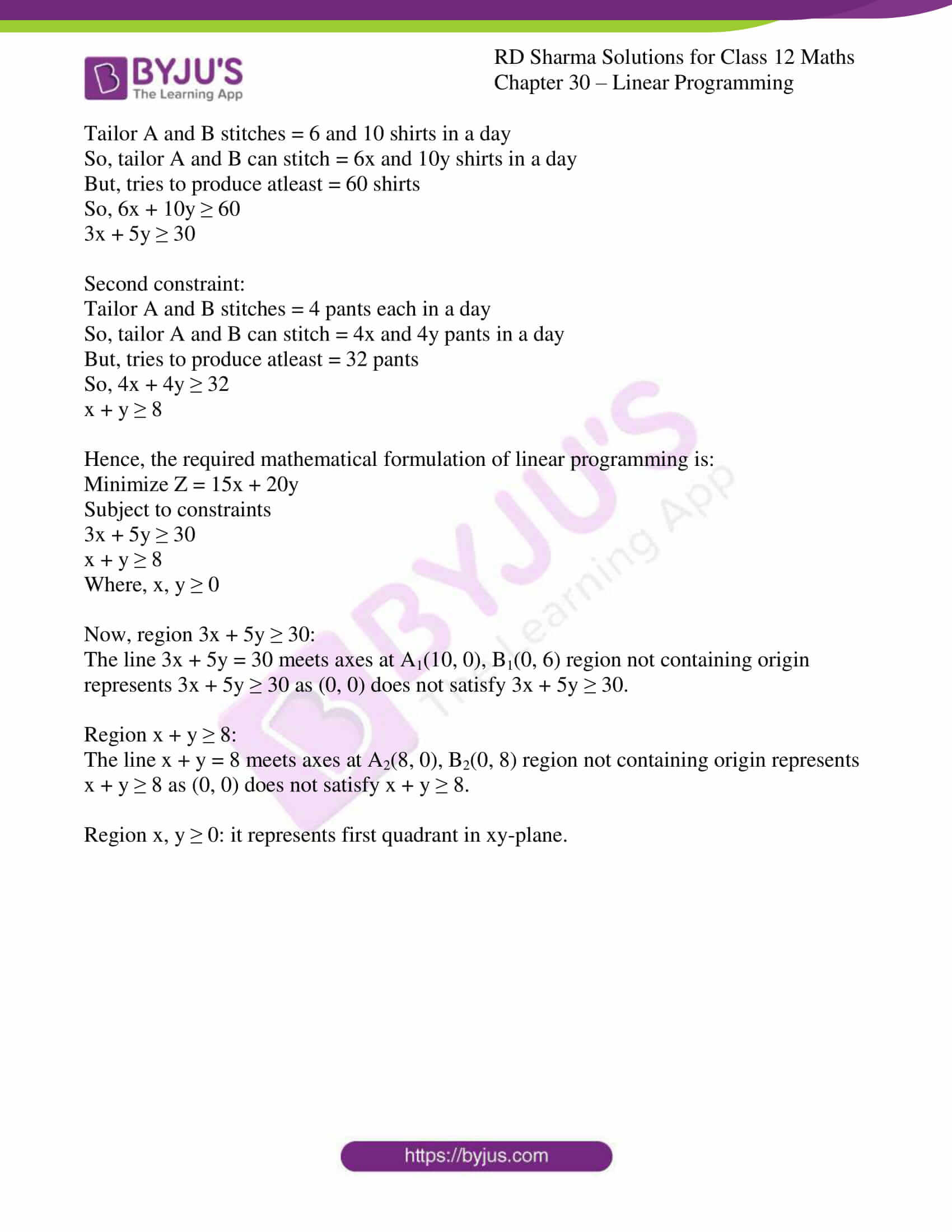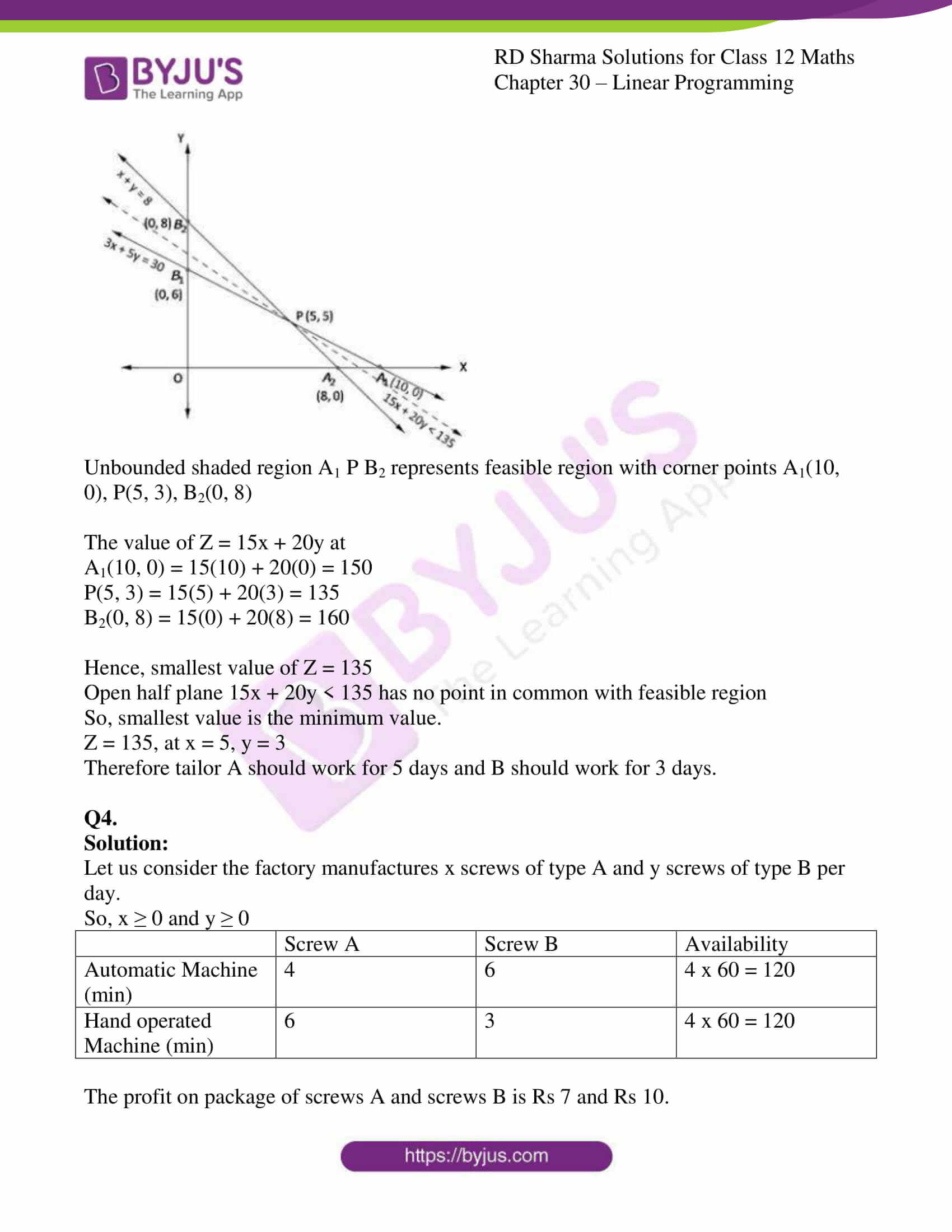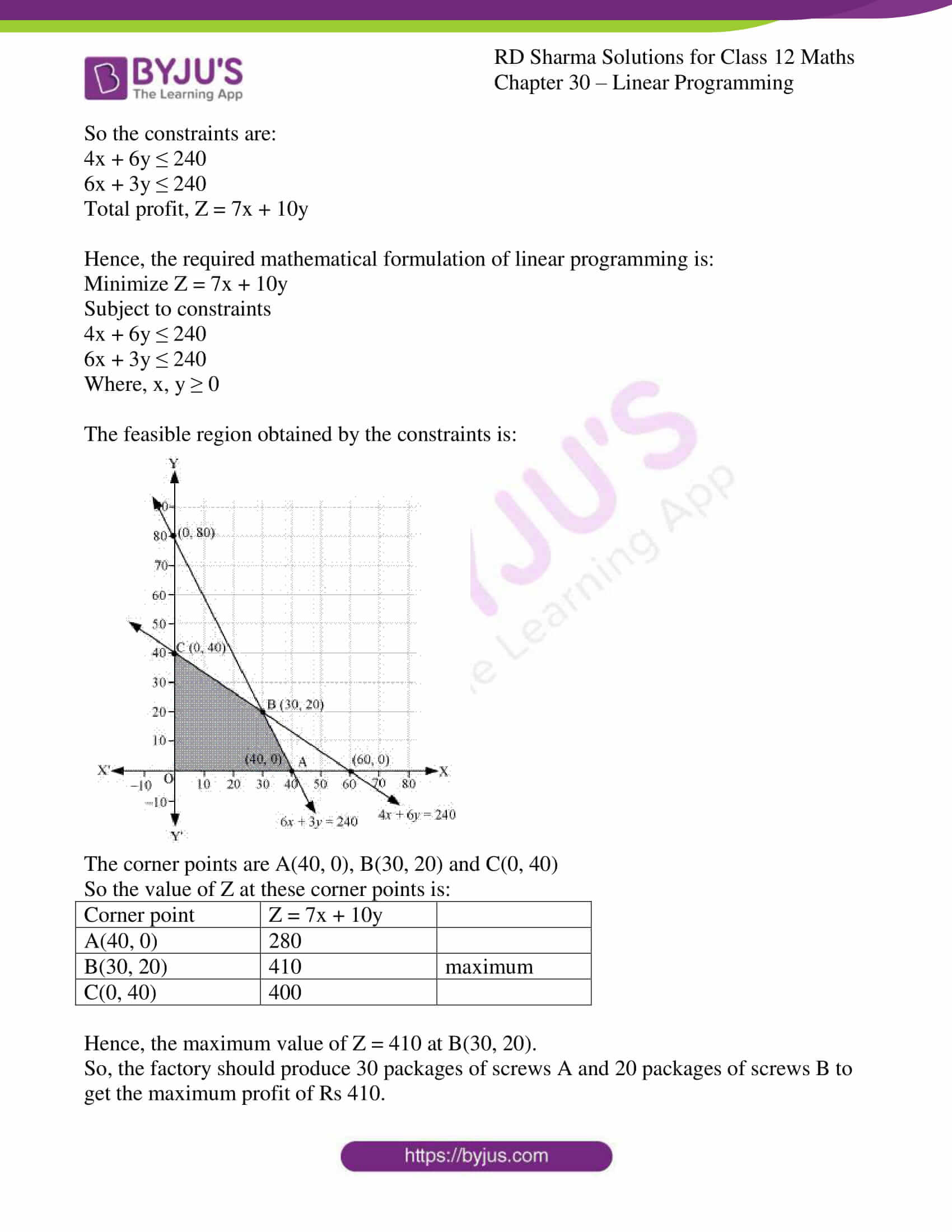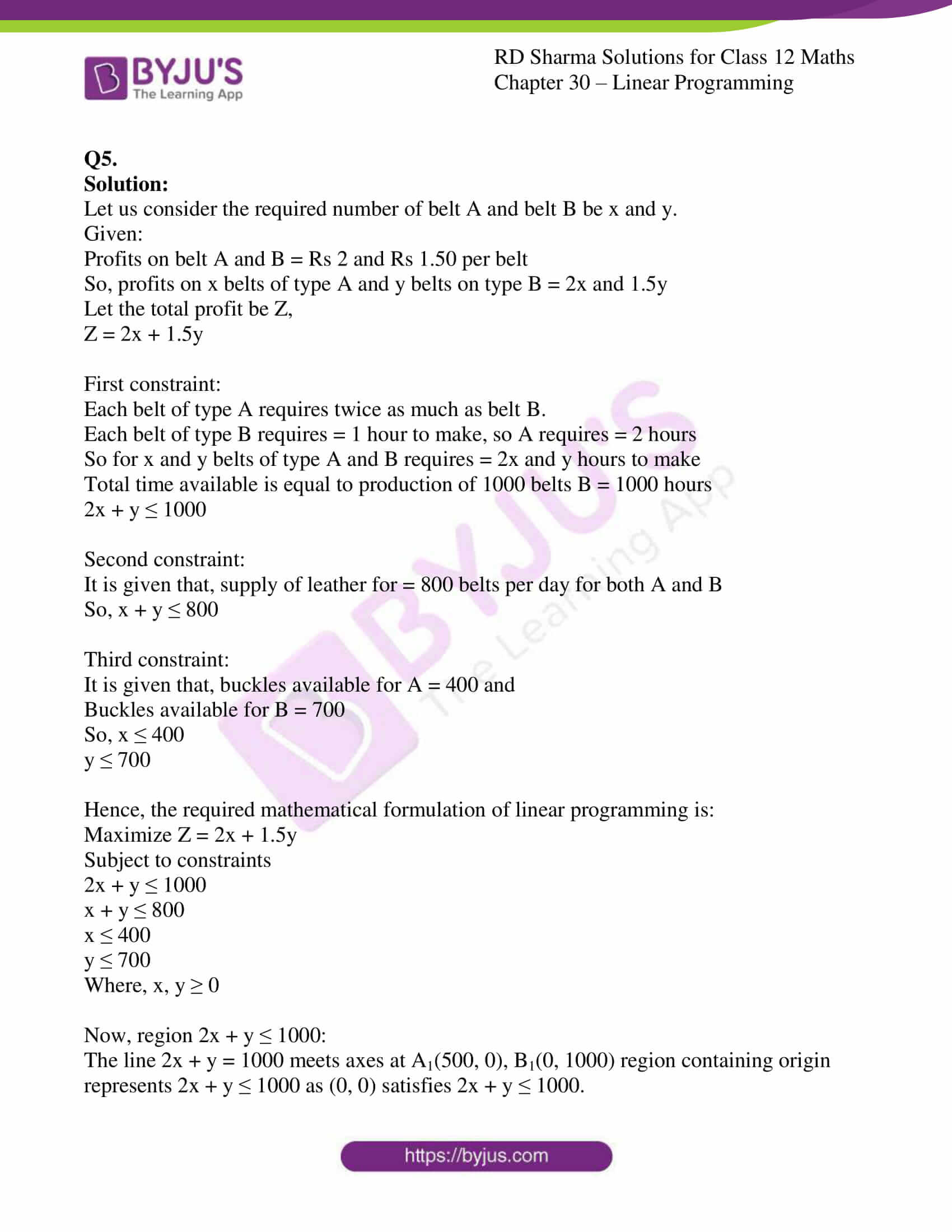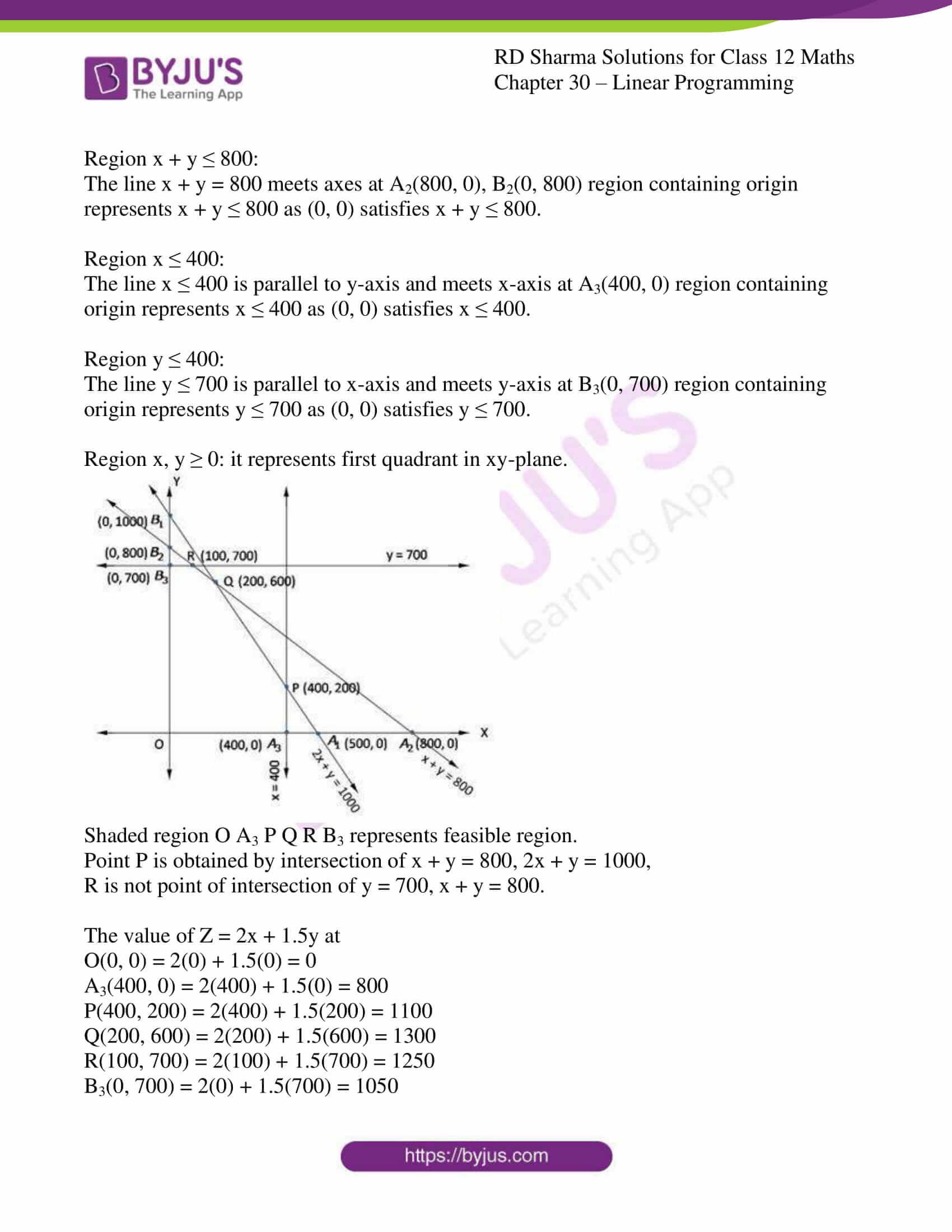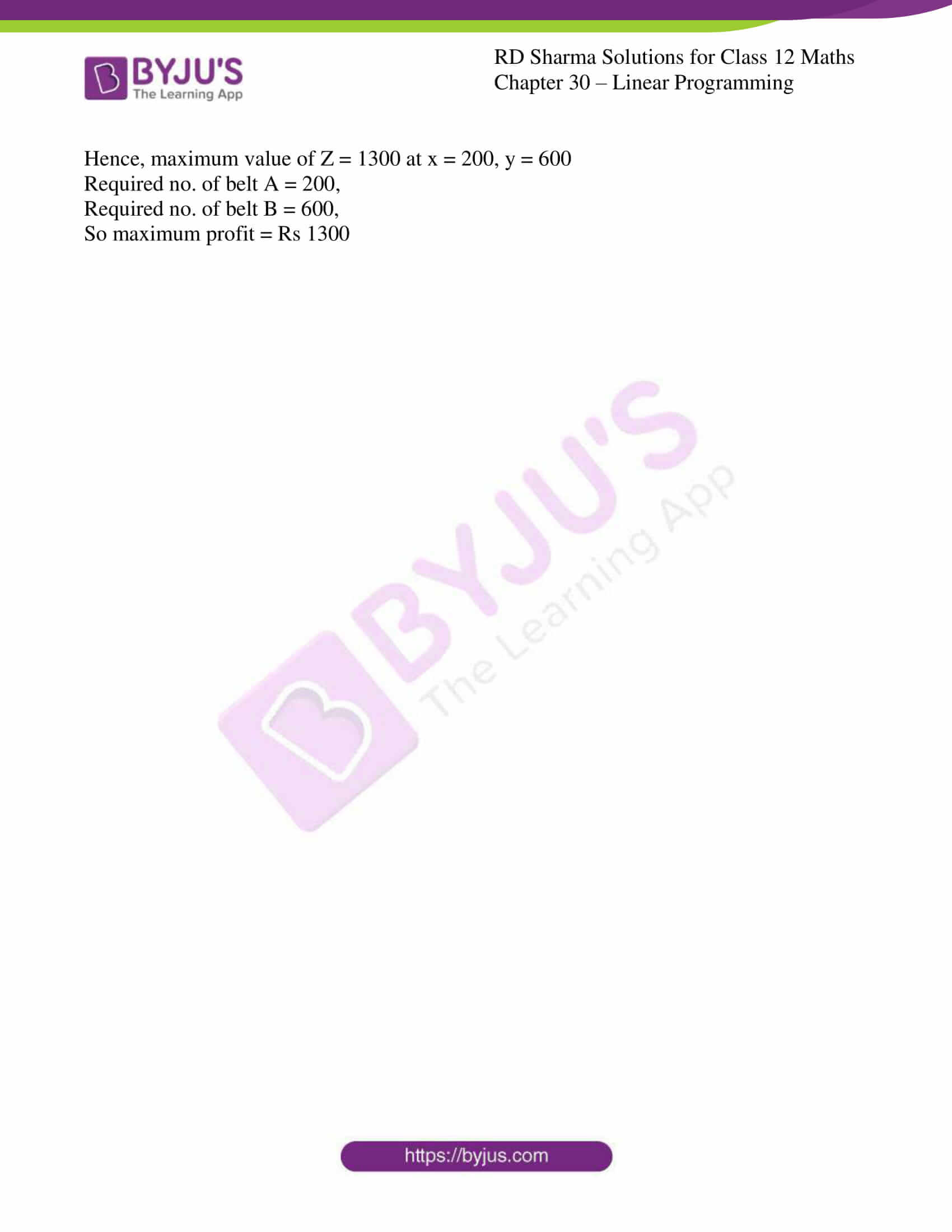### Access RD Sharma Solutions for Class 12 Maths Chapter 30 Exercise 4

EXERCISE 30.4

Q1.

Solution:

Let us consider a young man drive x km at a speed of 25km/hr and y km at a speed of 40km/hr.

Let Z be the total distance travelled by young man.

So, Z = x + y

First constraint:

When speed is 25km/hr, young man spends = Rs 2 per km

When speed is 40km/hr, young man spends = Rs 5 per km

So,

Expenses on x km and y km = Rs 2x and Rs 5y

But young man has only Rs 100

So, 2x + 5y ≤ 100

Second constraint:

Time taken to travel x km = Distance/speed

= x/25 hr

Time taken to travel y km = Distance/speed

= y/40 hr

It is given that, 1hr to travel,

So, x/25 + y/40 ≤ 1

40x + 25y ≤ 1000

8x + 5y ≤ 200

Hence, the required mathematical formulation of linear programming is:

Minimize Z = x + y

Subject to constraints

2x + 5y ≤ 100

8x + 5y ≤ 200

Where, x, y ≥ 0

Now, region 2x + 5y ≤ 100:

The line 2x + 5y = 100 meets axes at A1(50, 0), B1(0, 20) region containing origin represents 2x + 5y ≤ 100 as (0, 0) satisfies 2x + 5y ≤ 100.

Region 8x + 5y ≤ 200:

The line 8x + 5y = 200 meets axes at A2(25, 0), B2(0, 40) region containing origin represents 8x + 5y ≤ 200 as (0, 0) satisfies 8x + 5y ≤ 200.

Region x, y ≥ 0: it represents first quadrant in xy-plane.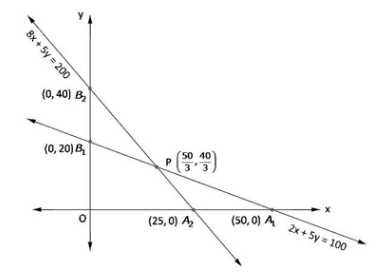Shaded region O A2 P B1 represents feasible region.

Point P(50/3, 40/3) is obtained by solving 8x + 5y = 200, 2x + y = 100

The value of Z = x + y at

O(0, 0) = 0 + 0 = 0

A2(25, 0) = 25 + 0 = 25

P(50/3, 40/3) = 50/3 + 40/3 = 30

B1(0, 20) = 0 + 20 = 20

Hence, maximum value of Z = 30 at x = 50/3, y = 40/3

Distance travelled at speed of 25km/hr = 50/3 km

And at speed of 40 km/hr = 40/3 km

So maximum distance = 30 km

Q2.

Solution:

Let us consider the required quantity of items be A and B.

Given:

Profits on one item A and B = Rs 6 and Rs 4

So, profits on x items of type A and y items on type B = 6x and 4y

Let the total profit be Z,

Z = 6x + 4y

First constraint:

Machine I works 1 hour and 2hours on item A and B

So, x no. of item A and y no. of item B = x hour and 2y hours on machine I

Machine I works at most = 12 hours

x + 2y ≥ 12

Second constraint:

Machine II works 2 hours and 1hour on item A and B

So, x no. of item A and y no. of item B = 2x hours and y hours on machine II

Machine II works maximum of = 12 hours

2x + y ≥ 12

Third constraint:

Machine III works 1 hour and 5/4hour on item A and B

So, x no. of item A and y no. of item B = x hours and 5/4y hours on machine III

Machine III works at least = 5 hours

x + 5/4y ≥ 5

4x + 5y ≥ 20

Hence, the required mathematical formulation of linear programming is:

Minimize Z = 6x + 4y

Subject to constraints

x + 2y ≥ 12

2x + y ≥ 12

4x + 5y ≥ 20

Where, x, y ≥ 0

Now, region x + 2y ≥ 12:

The line x + 2y = 12 meets axes at A1(12, 0), B1(0, 6) region containing origin represents x + 2y ≥ 12 as (0, 0) satisfies x + 2y ≥ 12.

Region 2x + y ≥ 12:

The line 2x + y = 12 meets axes at A1(6, 0), B1(0, 12) region containing origin represents 2x + y ≥ 12 as (0, 0) satisfies 2x + y ≥ 12.

Region 4x + 5y ≥ 20:

The line 4x + 5y = 20 meets axes at A3(5, 0), B3(0, 4) region not containing origin represents 4x + 5y ≥ 20 as (0, 0) does not satisfy 4x + 5y ≥ 20.

Region x, y ≥ 0: it represents first quadrant in xy-plane.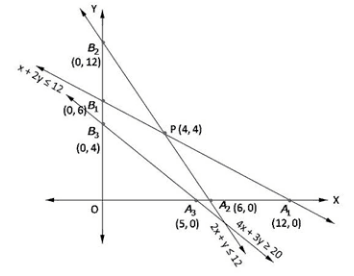Shaded region A2 A3 P B3 B1 represents feasible region.

The value of Z = 6x + 4y at

A2(6, 0) = 6(6) + 4(0) = 36

A3(5, 0) = 6(5) + 4(0) = 30

B3(0, 4) = 6(0) + 4(4) = 16

B2(0, 6) = 6(0) + 4(6) = 24

P(4,4) = 6(4) + 4(4) = 40

Hence, maximum value of Z = 40 at x = 4, y = 4

Required number of product A =4, product B = 4

So maximum profit = Rs 40

Q3.

Solution:

Let us consider tailor A and B work for x and y days.

Given:

Tailor A and B earn = Rs 15 and Rs 20

So, tailor A and B earn is x and y days = Rs 15x and 20y

Let the total maximum profit be Z which gives minimum labour cost,

Z = 15x + 20y

First constraint:

Tailor A and B stitches = 6 and 10 shirts in a day

So, tailor A and B can stitch = 6x and 10y shirts in a day

But, tries to produce atleast = 60 shirts

So, 6x + 10y ≥ 60

3x + 5y ≥ 30

Second constraint:

Tailor A and B stitches = 4 pants each in a day

So, tailor A and B can stitch = 4x and 4y pants in a day

But, tries to produce atleast = 32 pants

So, 4x + 4y ≥ 32

x + y ≥ 8

Hence, the required mathematical formulation of linear programming is:

Minimize Z = 15x + 20y

Subject to constraints

3x + 5y ≥ 30

x + y ≥ 8

Where, x, y ≥ 0

Now, region 3x + 5y ≥ 30:

The line 3x + 5y = 30 meets axes at A1(10, 0), B1(0, 6) region not containing origin represents 3x + 5y ≥ 30 as (0, 0) does not satisfy 3x + 5y ≥ 30.

Region x + y ≥ 8:

The line x + y = 8 meets axes at A2(8, 0), B2(0, 8) region not containing origin represents x + y ≥ 8 as (0, 0) does not satisfy x + y ≥ 8.

Region x, y ≥ 0: it represents first quadrant in xy-plane.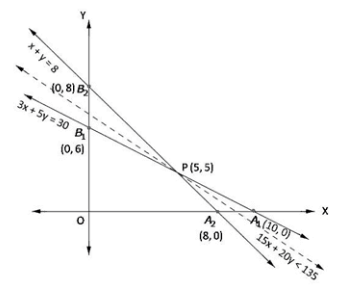Unbounded shaded region A1 P B2 represents feasible region with corner points A1(10, 0), P(5, 3), B2(0, 8)

The value of Z = 15x + 20y at

A1(10, 0) = 15(10) + 20(0) = 150

P(5, 3) = 15(5) + 20(3) = 135

B2(0, 8) = 15(0) + 20(8) = 160

Hence, smallest value of Z = 135

Open half plane 15x + 20y < 135 has no point in common with feasible region

So, smallest value is the minimum value.

Z = 135, at x = 5, y = 3

Therefore tailor A should work for 5 days and B should work for 3 days.

Q4.

Solution:

Let us consider the factory manufactures x screws of type A and y screws of type B per day.

So, x ≥ 0 and y ≥ 0

 Screw A Screw B Availability Automatic Machine (min) 4 6 4 x 60 = 120 Hand operated Machine (min) 6 3 4 x 60 = 120

The profit on package of screws A and screws B is Rs 7 and Rs 10.

So the constraints are:

4x + 6y ≤ 240

6x + 3y ≤ 240

Total profit, Z = 7x + 10y

Hence, the required mathematical formulation of linear programming is:

Minimize Z = 7x + 10y

Subject to constraints

4x + 6y ≤ 240

6x + 3y ≤ 240

Where, x, y ≥ 0

The feasible region obtained by the constraints is: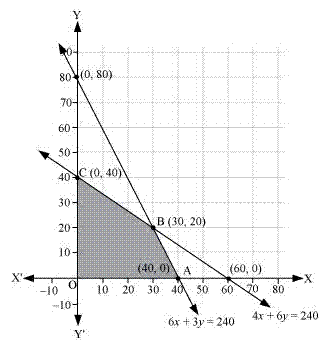The corner points are A(40, 0), B(30, 20) and C(0, 40)

So the value of Z at these corner points is:

 Corner point Z = 7x + 10y A(40, 0) 280 B(30, 20) 410 maximum C(0, 40) 400

Hence, the maximum value of Z = 410 at B(30, 20).

So, the factory should produce 30 packages of screws A and 20 packages of screws B to get the maximum profit of Rs 410.

Q5.

Solution:

Let us consider the required number of belt A and belt B be x and y.

Given:

Profits on belt A and B = Rs 2 and Rs 1.50 per belt

So, profits on x belts of type A and y belts on type B = 2x and 1.5y

Let the total profit be Z,

Z = 2x + 1.5y

First constraint:

Each belt of type A requires twice as much as belt B.

Each belt of type B requires = 1 hour to make, so A requires = 2 hours

So for x and y belts of type A and B requires = 2x and y hours to make

Total time available is equal to production of 1000 belts B = 1000 hours

2x + y ≤ 1000

Second constraint:

It is given that, supply of leather for = 800 belts per day for both A and B

So, x + y ≤ 800

Third constraint:

It is given that, buckles available for A = 400 and

Buckles available for B = 700

So, x ≤ 400

y ≤ 700

Hence, the required mathematical formulation of linear programming is:

Maximize Z = 2x + 1.5y

Subject to constraints

2x + y ≤ 1000

x + y ≤ 800

x ≤ 400

y ≤ 700

Where, x, y ≥ 0

Now, region 2x + y ≤ 1000:

The line 2x + y = 1000 meets axes at A1(500, 0), B1(0, 1000) region containing origin represents 2x + y ≤ 1000 as (0, 0) satisfies 2x + y ≤ 1000.

Region x + y ≤ 800:

The line x + y = 800 meets axes at A2(800, 0), B2(0, 800) region containing origin represents x + y ≤ 800 as (0, 0) satisfies x + y ≤ 800.

Region x ≤ 400:

The line x ≤ 400 is parallel to y-axis and meets x-axis at A3(400, 0) region containing origin represents x ≤ 400 as (0, 0) satisfies x ≤ 400.

Region y ≤ 400:

The line y ≤ 700 is parallel to x-axis and meets y-axis at B3(0, 700) region containing origin represents y ≤ 700 as (0, 0) satisfies y ≤ 700.

Region x, y ≥ 0: it represents first quadrant in xy-plane.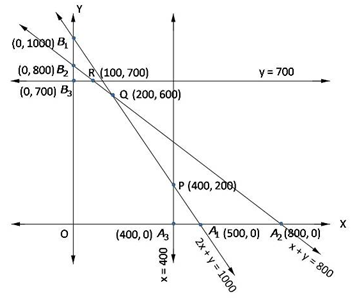Shaded region O A3 P Q R B3 represents feasible region.

Point P is obtained by intersection of x + y = 800, 2x + y = 1000,

R is not point of intersection of y = 700, x + y = 800.

The value of Z = 2x + 1.5y at

O(0, 0) = 2(0) + 1.5(0) = 0

A3(400, 0) = 2(400) + 1.5(0) = 800

P(400, 200) = 2(400) + 1.5(200) = 1100

Q(200, 600) = 2(200) + 1.5(600) = 1300

R(100, 700) = 2(100) + 1.5(700) = 1250

B3(0, 700) = 2(0) + 1.5(700) = 1050

Hence, maximum value of Z = 1300 at x = 200, y = 600

Required no. of belt A = 200,

Required no. of belt B = 600,

So maximum profit = Rs 1300